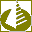Mathematics

# Mathematics

Sort by:
Results: 1-3 of 3Nonlinear analysis - DataFitting 1.7.63
DataFitting is a powerful statistical analysis program that performs linear and nonlinear regression analysis (i.e. curve fitting).
Shareware, Updated 5 February 2023
User rating
932
Last week
7
updated

1.29 MBEquation graph plotter - EqPlot 1.3.57
EqPlot plots 2D graphs from complex equations. The application comprises algebraic, trigonometric, hyperbolic and transcendental functions. EqPlot can be used to verify the results of nonlinear regression analysis program.
Shareware, Updated 6 February 2023
User rating
1,680
Last week
3
updated

1.40 MBNonlinear regression - CurveFitter 4.5.61
CurveFitter performs statistical regression analysis to estimate the values of parameters for linear, multivariate, polynomial, exponential and nonlinear functions.
Shareware, Updated 6 February 2023
User rating